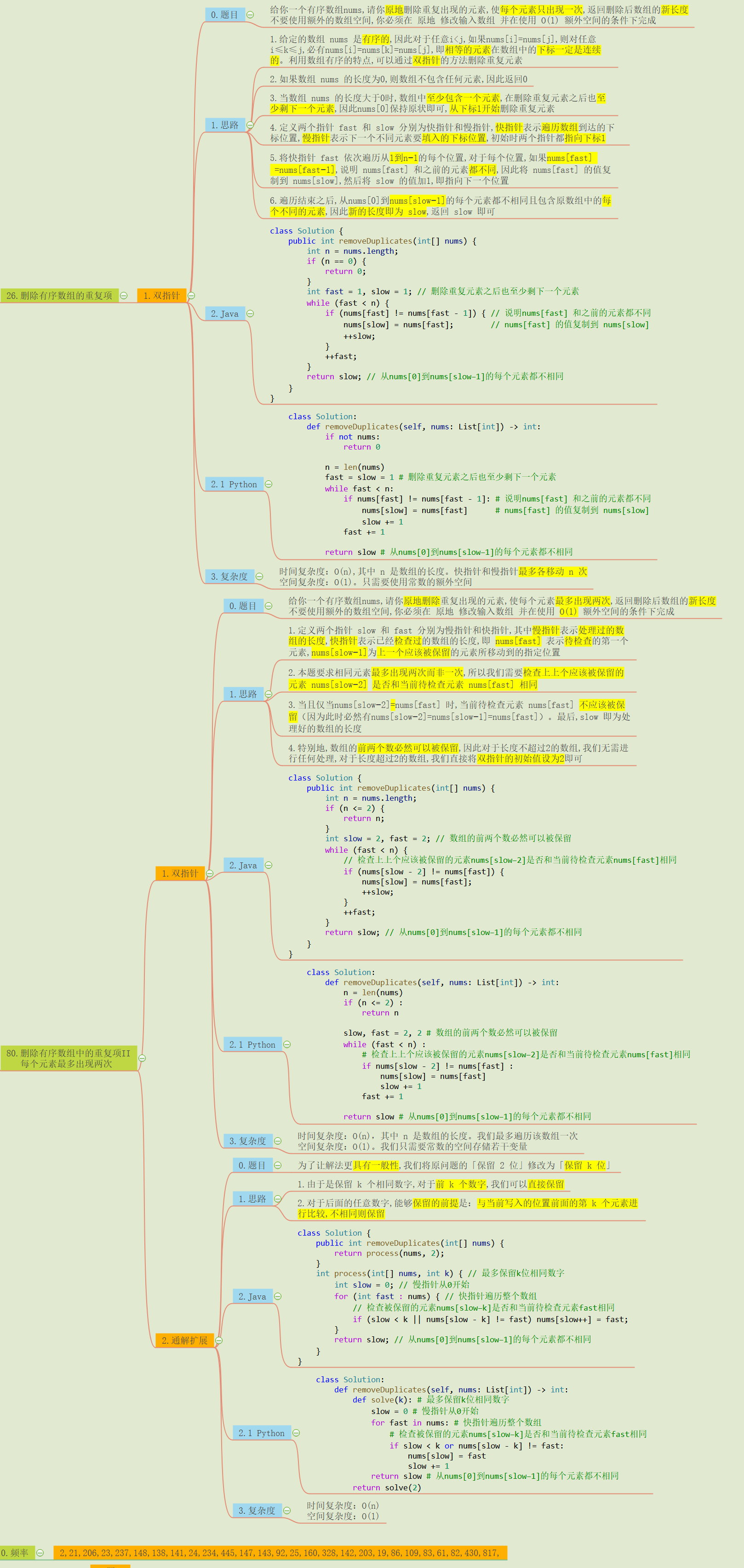# ❤️思维导图整理大厂面试高频数组9: 删除重复元素的通解问题, 力扣26/80❤️

1月前   阅读数 44 0

### 文章目录

https://leetcode-cn.com/problems/remove-duplicates-from-sorted-array-ii/

## 0.导图整理## 源码

### Python:

``````# 删除有序数组的重复项
class Solution:
def removeDuplicates(self, nums: List[int]) -> int:
if not nums:
return 0

n = len(nums)
fast = slow = 1 # 删除重复元素之后也至少剩下一个元素
while fast < n:
if nums[fast] != nums[fast - 1]: # 说明nums[fast] 和之前的元素都不同
nums[slow] = nums[fast]      # nums[fast] 的值复制到 nums[slow]
slow += 1
fast += 1

return slow # 从nums到nums[slow−1]的每个元素都不相同

# 删除有序数组中的重复项II 每个元素最多出现两次
class Solution:
def removeDuplicates(self, nums: List[int]) -> int:
n = len(nums)
if (n <= 2) :
return n

slow, fast = 2, 2 # 数组的前两个数必然可以被保留
while (fast < n) :
# 检查上上个应该被保留的元素nums[slow−2]是否和当前待检查元素nums[fast]相同
if nums[slow - 2] != nums[fast] :
nums[slow] = nums[fast]
slow += 1
fast += 1

return slow # 从nums到nums[slow−1]的每个元素都不相同

# 通解扩展
class Solution:
def removeDuplicates(self, nums: List[int]) -> int:
def solve(k): # 最多保留k位相同数字
slow = 0 # 慢指针从0开始
for fast in nums: # 快指针遍历整个数组
# 检查被保留的元素nums[slow−k]是否和当前待检查元素fast相同
if slow < k or nums[slow - k] != fast:
nums[slow] = fast
slow += 1
return slow # 从nums到nums[slow−1]的每个元素都不相同
return solve(2)
``````

### java:

``````// 删除有序数组的重复项
class Solution {

public int removeDuplicates(int[] nums) {

int n = nums.length;
if (n == 0) {

return 0;
}
int fast = 1, slow = 1; // 删除重复元素之后也至少剩下一个元素
while (fast < n) {

if (nums[fast] != nums[fast - 1]) {
// 说明nums[fast] 和之前的元素都不同
nums[slow] = nums[fast];        // nums[fast] 的值复制到 nums[slow]
++slow;
}
++fast;
}
return slow; // 从nums到nums[slow−1]的每个元素都不相同
}
}

// 删除有序数组中的重复项II 每个元素最多出现两次
class Solution {

public int removeDuplicates(int[] nums) {

int n = nums.length;
if (n <= 2) {

return n;
}
int slow = 2, fast = 2; // 数组的前两个数必然可以被保留
while (fast < n) {

// 检查上上个应该被保留的元素nums[slow−2]是否和当前待检查元素nums[fast]相同
if (nums[slow - 2] != nums[fast]) {

nums[slow] = nums[fast];
++slow;
}
++fast;
}
return slow; // 从nums到nums[slow−1]的每个元素都不相同
}
}

// 通解扩展
class Solution {

public int removeDuplicates(int[] nums) {

return process(nums, 2);
}
int process(int[] nums, int k) {
// 最多保留k位相同数字
int slow = 0; // 慢指针从0开始
for (int fast : nums) {
// 快指针遍历整个数组
// 检查被保留的元素nums[slow−k]是否和当前待检查元素fast相同
if (slow < k || nums[slow - k] != fast) nums[slow++] = fast;
}
return slow; // 从nums到nums[slow−1]的每个元素都不相同
}
}
``````

Python相关技术 知识点 思维导图整理

Java相关技术/ssm框架全部笔记

我有话说: